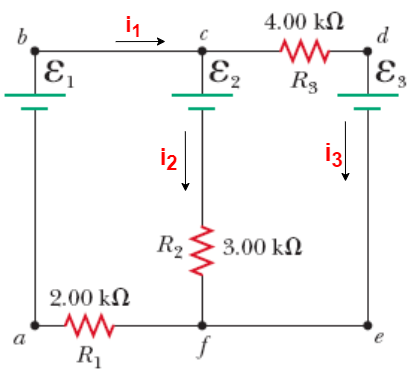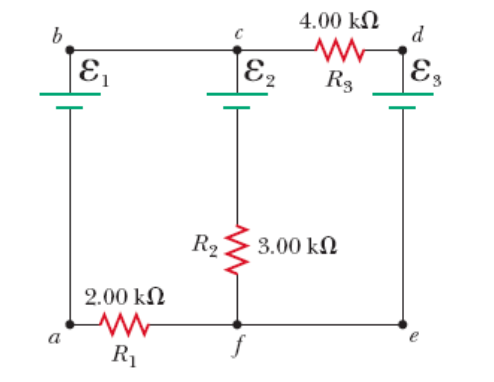# Problem: Using Kirchhoff’s rules (and given ε1 = 70.7 V, ε2 = 61.4 V, ε3 = 79.9 V) calculate the following:(a) Find the current in each resistor shown in the figure above.(b) Find the potential difference between points c and f.(c) Which point is at higher potential?a. cb. f

###### FREE Expert Solution

Kirchhoff's loop rule:

$\overline{){\mathbf{\Sigma }}{\mathbf{V}}{\mathbf{=}}{\mathbf{0}}}$

Kirchhoff's junction rule:

$\overline{){\mathbf{\Sigma }}{{\mathbf{i}}}_{\mathbf{i}\mathbf{n}}{\mathbf{=}}{\mathbf{\Sigma }}{{\mathbf{i}}}_{\mathbf{o}\mathbf{u}\mathbf{t}}}$

We'll assign current directions to the diagram. The junctions are already labeled.(a)

At junction c:

i1 = i2 + i3

In the circuit, we have 3 unknowns (the currents only). We need an expression to substitute for one of the currents so that we remain with only 2 unknowns when working out Kirchhoff's loop rule.

Solving for i2:

i2 = i1 - i3

We'll proceed to Kirchoff's loop rule. With only two unknowns, we'll consider any two loops. We have three loops: Left, right, and outer loop.

80% (55 ratings)###### Problem Details

Using Kirchhoff’s rules (and given ε1 = 70.7 V, ε2 = 61.4 V, ε3 = 79.9 V) calculate the following:(a) Find the current in each resistor shown in the figure above.
(b) Find the potential difference between points c and f.
(c) Which point is at higher potential?
a. c
b. f